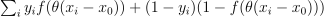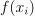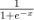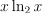# module ml.logreg#

## Short summary#

module mlstatpy.ml.logreg

Helpers on logistic regression.

source on GitHub

## Functions#

function

truncated documentation

criteria

Computes Gini, information gain, likelihood on a dataset with two features assuming the first coordinates is used to …

criteria2

Computes Gini, information gain, likelihood on a dataset with two features assuming the first coordinates is used to …

likelihood

Computeswhereis. …

logistic

Computes.

plog2

Computes.

plot_ds

Plots a dataset, X is a dataset with two features, y contains the binary labels.

random_set_1d

Builds a random dataset as describes in example Arbre d’indécision.

## Documentation#

Helpers on logistic regression.

source on GitHub

mlstatpy.ml.logreg.criteria(X, y)#

Computes Gini, information gain, likelihood on a dataset with two features assuming the first coordinates is used to classify.

Paramètres:
• X – 2D matrix

• y – binary labels

Renvoie:

dataframe

source on GitHub

mlstatpy.ml.logreg.criteria2(X, y)#

Computes Gini, information gain, likelihood on a dataset with two features assuming the first coordinates is used to classify.

Paramètres:
• X – 2D matrix

• y – binary labels

Renvoie:

dataframe

source on GitHub

mlstatpy.ml.logreg.likelihood(x, y, theta=1.0, th=0.0)#

Computeswhereis.

source on GitHub

mlstatpy.ml.logreg.logistic(x)#

Computes.

source on GitHub

mlstatpy.ml.logreg.plog2(p)#

Computes.

source on GitHub

mlstatpy.ml.logreg.plot_ds(X, y, ax=None, title=None)#

Plots a dataset, X is a dataset with two features, y contains the binary labels.

source on GitHub

mlstatpy.ml.logreg.random_set_1d(n, kind)#

Builds a random dataset as describes in example Arbre d’indécision.

Paramètres:
• n – number of observations

• kind – 2, 3, 4 (see example)

Renvoie:

array 2D

source on GitHub